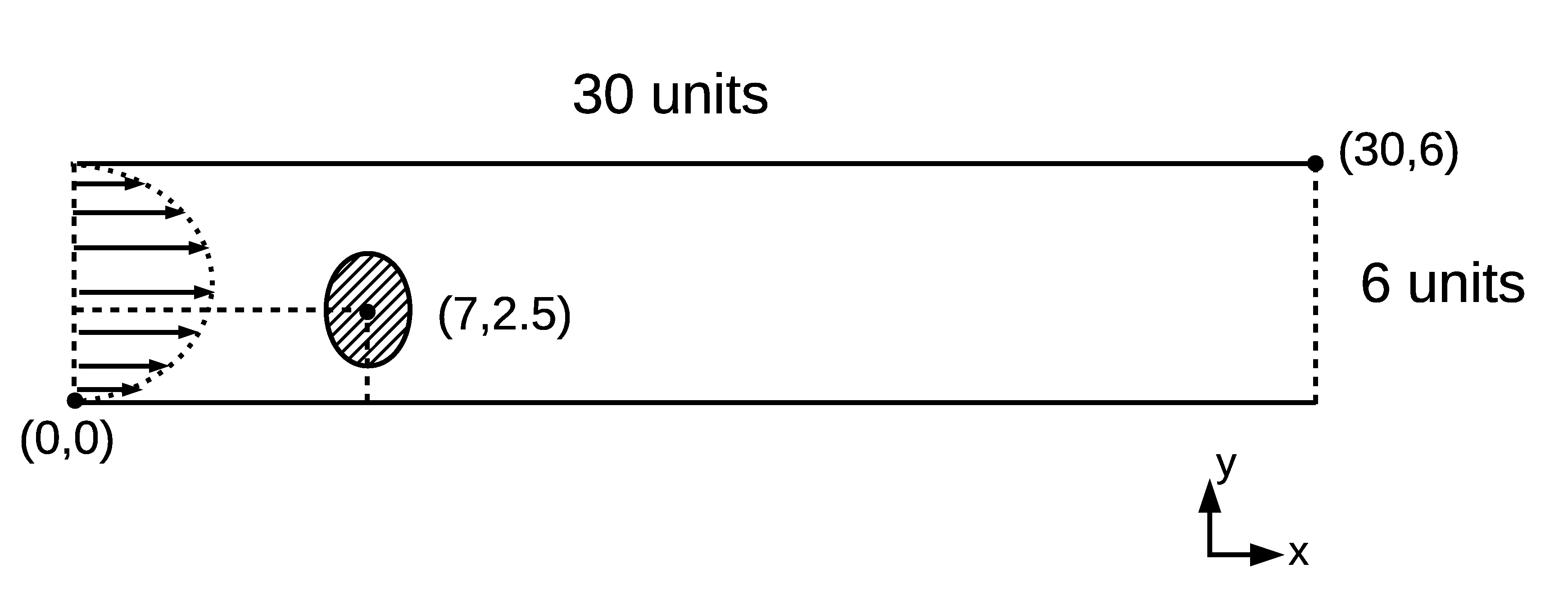In :
from IPython.display import HTML

HTML('''<script>
code_show=true;
function code_toggle() {
if (code_show){
$('div.input').hide();$('div.prompt').hide();
} else {
$('div.input').show();$('div.prompt').show();
}
code_show = !code_show
}
$( document ).ready(code_toggle); </script> <form action="javascript:code_toggle()"><input type="submit" value="Code Toggle"></form>''')  Out: In : %%javascript MathJax.Hub.Config({ TeX: { equationNumbers: { autoNumber: "AMS" } } });  In : from IPython.display import HTML HTML(''' <a href="https://raw.githubusercontent.com/usnistgov/pfhub/master/benchmarks/benchmark5-hackathon.ipynb" download="benchmark5-hackathon.ipynb"> <button type="submit">Download Notebook</button> </a>''')  Out: # Benchmark Problem 5: Stokes Flow¶ In : from IPython.display import HTML HTML('''{% include jupyter_benchmark_table.html num="" revision=0 %}''')  Out: ##### Revision History See the full benchmark table and table key See the Overleaf document entitled "Phase Field Benchmark Problems for Dendritic Growth and Linear Elasticity" for more details about the benchmark problems. Furthermore, read the extended essay for a discussion about the need for benchmark problems. # Overview¶ Flow of a liquid can be incorporated into phase field models, so we present this benchmark problem for incompressible fluid flow through a channel (the flow of many liquids can be modeled as incompressible). The flow of a fluid can generally be modeled via the Navier-Stokes equations. When length scales are small, fluid velocities are low, and/or viscosity is large, such that the Reynolds number$Re<<1$, inertial forces are small compared with viscous forces, resulting in a simplification of Navier-Stokes flow to Stokes flow. # Model Formulation¶ ## Governing Equations¶ In this problem, two variables are used: the fluid velocity,$\textbf{u}$, which is a vector field, and the fluid pressure,$p$, which is a scalar field. The Stokes momentum equation is given as \begin{equation} -\mu \nabla^{2} \textbf{u} + \nabla p - \rho \textbf{g} = 0, \end{equation} where$\rho$is the density, assumed constant in this problem,$\mu$is the dynamic viscosity, and$\textbf{g}$is the acceleration due to gravity. To fully describe fluid flow, the momentum balance equation is supplemented with the continuity equation for mass flow, \begin{equation} \frac{d\rho}{dt}+\nabla\cdot\left(\rho{\mathbf u}\right)=0; \end{equation} this simplifies to \begin{equation} \nabla \cdot \textbf{u} = 0 \end{equation} for incompressible flow. Use$\rho=100$,$\mu=1$, and$\textbf{g}=(0,-0.001)$. ## Domain¶ In this problem, we consider flow in a 2D channel (a) without and (b) with an obstruction. The computational domain for case (b) is shown below with inlet boundary condition indicated by arrows for the Stokes flow benchmark problem with an obstruction (case (b)). The domain and boundary conditions, etc., for case (a) are the same as that in case (b), but without the obstruction. ### Figure 1: Domain for variation (b)¶## Boundary Conditions¶ All solid surfaces, including the boundary for the obstruction, have no-slip boundary conditions, that is,$u_x=u_y=0$. The inlet velocity, on the left boundary, follows a parabolic profile described by \begin{equation} u_x(0,y) = -0.001(y-3)^2+0.009. \end{equation} The outlet velocity (on the right boundary) is left to the solver to determine, as is the pressure over the entire domain. However, we specify that the pressure at point (30, 6) is zero. Finally, the obstruction is described by an ellipse centered at (7, 2.5). The semi-major axis (in the y-direction) is$a=1.5$, and the semi-minor axis (in the x-direction) is$b=1$. ## Submission Guidelines¶ Both variation (a) and (b) should be run to steady state. Please submit the steady state pressure and the steady state velocity fields for both variation (a) and (b) along the$x=7$and$y=5\$ cut planes.

This will require two CSV or JSON files for each variation. Please,

• link to your first CSV or JSON file, labeled x_cut_plane in the upload form; the columns or keys should be named y, velocity_x, velocity_y and pressure

• link to your second CSV or JSON file, labeled y_cut_plane in the upload form; the columns or keys should be named x, velocity_x, velocity_y and pressure

Further data to upload can include images of the pressure and velocity fields at steady state. These are not required, but will help others view your work.

Upload Benchmark Results